Cognizant

3.9

At Cognizant, we give organizations the insights to anticipate what customers want and act instantly to deliver on those demands. So they can achieve the goal of every modern business: staying one step ahe... read moreTeaneck, New Jersey, United States (USA)Engaged employerFilter interviews by
Designation

Select Designation

Clear

1.4k results found

### Interview Rounds

Coding Test

Video Call

HR

I was interviewed in Sep, 2021.

### Interview Questions

• Q1. Buy and Sell Stock - III

#### You can perform a transition with these conditions -

``````1. Not allowed to engage in more than 1 transaction at a time, which means if you have bought stock then you can buy another stock before selling the first stock.

2. If you bought a stock at ‘X’ price and sold it at ‘Y’ price then the profits ‘Y - X’.
``````
##### Note:
``````It is not compulsory to perform an exact '2' transaction.
``````
##### Input format:
``````The first line of input contains an integer 'T' denoting the number of test cases.

The first line of each test case contains a single integer 'N', 'N' denotes the length of array ‘prices’.

The second line of each test case contains an 'N' space-separated integers, in which every integer denotes an element of the array "prices".
``````
##### Output Format
``````For each test case, you need to print the maximum profit made by selling the stocks.
``````
##### Note :
``````You do not need to print anything; it has already been taken care of.
``````
##### Constraints:
``````1 <= T <= 50
1 <= N <= 10^4
0 <= A[i] <= 10^9

Time limit: 1 second
``````
• Q2. Pairwise Sum of Hamming Distance

#### For example:

``````Consider A=4 and B=7
Binary representation of 4 = 100
Binary representation of 7 = 111
For the given example, if we flip the values of the last two least significant bits of A to 1 then A will become 7. As we can change the value of A to B by 2 flips. Therefore the Hamming Distance, in this case, is 2.
``````
##### Input format:
``````The first line of input contains an integer ‘T’ denoting the number of test cases.

The first line of each test case contains an integer ‘N’ denoting the number of elements in the array.

The second line of each test contains 'N' space-separated integers denoting the array elements.
``````
##### Output Format:
``````For each test case, return the sum of Hamming Distance for all the pairs.
``````
##### Note:
``````You are not required to print anything just implement the given function.
``````
##### Constraints:
``````1 <= T <= 10
1 <= N <= 10^4
0 <= ARR[i] <=10^9

Time limit: 1 second
``````
• Q3. Next Greater Element

#### For a given array/list of integers of size N, print the Next Greater Element(NGE) for every element. The Next Greater Element for an element X is the first element on the right side of X in the array, which is greater than X. If no greater elements exist to the right of X, consider the next greater element as -1.

##### For example:
``````For the given array [7, 12, 1, 20]

- The next greater element for 7 is 12.
- The next greater element for 12 is 20.
- The next greater element for 1 is 20.
- There is no greater element for 20 on the right side.

Output: [12, 20, 20, -1]
``````
##### Input Format:
``````The first line of input contains an integer 'N' representing the size of the array/list.

The second line of input contains 'N' single space-separated integers representing the elements of the array/list.
``````
##### Output Format :
``````The only line of output contains 'N' single space-separated integers representing the Next Greater Element for each element.
``````
##### Note :
``````You do not need to print anything explicitly, it has already been taken care of.
``````
##### Constraints :
``````1 <= N <= 10^5
1 <= ARR[i] <= 10^9

Time Limit: 1 sec
``````
• Q4. Left View Of Binary Tree

#### Given a binary tree. Print the Left View of the Tree.

##### Example :
``````If the input tree is as depicted in the picture:
``````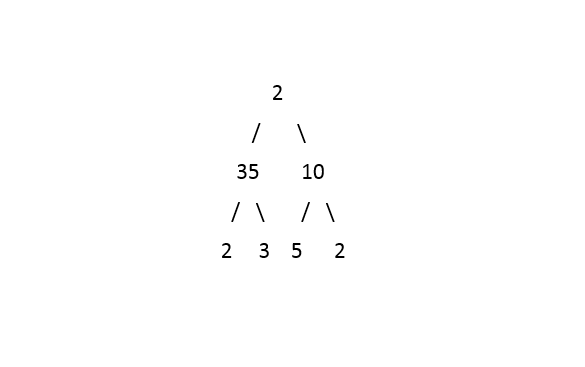``````The Left View of the tree will be:  2 35 2
``````
##### Input format :
``````Elements in the level order form. The input consists of values of nodes separated by a single space in a single line. In case a node is null, we take -1 in its place.

For example, the input for the tree depicted in the below image would be :
````````````1
2 3
4 -1 5 6
-1 7 -1 -1 -1 -1
-1 -1
``````

#### Explanation :

``````Level 1 :
The root node of the tree is 1

Level 2 :
Left child of 1 = 2
Right child of 1 = 3

Level 3 :
Left child of 2 = 4
Right child of 2 = null (-1)
Left child of 3 = 5
Right child of 3 = 6

Level 4 :
Left child of 4 = null (-1)
Right child of 4 = 7
Left child of 5 = null (-1)
Right child of 5 = null (-1)
Left child of 6 = null (-1)
Right child of 6 = null (-1)

Level 5 :
Left child of 7 = null (-1)
Right child of 7 = null (-1)

The first not-null node (of the previous level) is treated as the parent of the first two nodes of the current level. The second not-null node (of the previous level) is treated as the parent node for the next two nodes of the current level and so on.

The input ends when all nodes at the last level are null (-1).
``````
##### Note :
``````The above format was just to provide clarity on how the input is formed for a given tree.

The sequence will be put together in a single line separated by a single space. Hence, for the above-depicted tree, the input will be given as:

1 2 3 4 -1 5 6 -1 7 -1 -1 -1 -1 -1 -1
``````
##### Output Format :
``````The only line of output will print the data of in the Left View of the Tree separated by a single space.
``````
##### Constraints :
``````0 <= N <= 10^5
1 <= data <= 10^5

Where ‘N’ is the total number of nodes in the binary tree, and 'data' is the value of the binary tree node.

Time limit: 1sec
``````
• Q5. Basic HR Questions

Show me your id card
Relocation related questions
Which tech you are using
Some questions around projects
What's the famous food in the ongole ?
Who is your favorite hero ?

### Interview details

Professional and academic backgroundI completed Computer Science Engineering from Godavari Institute of Engineering and Technology. I applied for the job as Big data Analyst in HyderabadProposed: Eligibility criteria65% above (No backlogs)
Cognizant interview Rounds:Round 1
Round type - Online Coding Interview
Round duration - 180 minutes
Round difficulty - Easy

Round 2
Round type - Video Call
Round duration - 30 minnutes
Round difficulty - Hard

Round 3
Round type - HR Round
Round duration - 10 minutes
Round difficulty - Medium
Cognizant interview preparation:Topics to prepare for the interview - Python, DSA, Aptitude, SQL, Html, css, javascriptTime required to prepare for the interview - 1 monthInterview preparation tips for other job seekers

Tip 1 : practice more on programming
Tip 2 : just first learn basics and go for advance
Tip 3 : the questions on database are more important that can increases more package just well prepare on sql

Application resume tips for other job seekers

Tip 1 : everything should be format in a nice way don't add colors to resume keep it simple
Tip 2 : first name-->address->education->experience->projects/open source->achievements

Final outcome of the interviewRejected
View More2

### Interview Rounds

Coding Test

HR

I was interviewed in Nov, 2020.

### Interview Questions

• Q1. Merge Intervals

#### For example:

``````For the given 5 intervals - [1, 4], [3, 5], [6, 8], [10, 12], [8, 9].

Since intervals [1, 4] and [3, 5] overlap with each other, we will merge them into a single interval as [1, 5].

Similarly, [6, 8] and [8, 9] overlap, merge them into [6,9].

Interval [10, 12] does not overlap with any interval.

Final List after merging overlapping intervals: [1, 5], [6, 9], [10, 12].
``````
##### Input Format:
``````The first line of input contains an integer N, the number of intervals.

The second line of input contains N integers, i.e. all the start times of the N intervals.

The third line of input contains N integers, i.e. all the end times of the N intervals.
``````
##### Output Format:
``````Print S lines, each contains two single space-separated integers A, and B, where S is the size of the merged array of intervals, 'A' is the start time of an interval and 'B' is the end time of the same interval.
``````

#### Note

``````You do not need to print anything, it has already been taken care of. Just implement the given function.
``````
##### Constraints:
``````1 <= N <= 10^5
0 <= START, FINISH <= 10^9

Time Limit: 1sec
``````
• Q2. Bottom view of binary tree

#### A node will be in the bottom-view if it is the bottom-most node at its horizontal distance from the root.

##### Note :
``````1. The horizontal distance of the root from itself is 0. The horizontal distance of the right child of the root node is 1 and the horizontal distance of the left child of the root node is -1.

2. The horizontal distance of node 'n' from root = horizontal distance of its parent from root + 1, if node 'n' is the right child of its parent.

3. The horizontal distance of node 'n' from root = horizontal distance of its parent from the root - 1, if node 'n' is the left child of its parent.

4. If more than one node is at the same horizontal distance and is the bottom-most node for that horizontal distance, including the one which is more towards the right.

5. You are not required to print the output explicitly, it has already been taken care of. Just implement the function and return the answer, which is the sequence of nodes from left to right in the bottom view.
``````

For example, for the given Binary Tree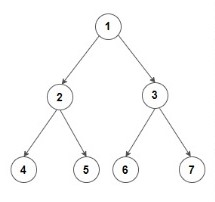``````Below is the bottom view of the binary tree.
``````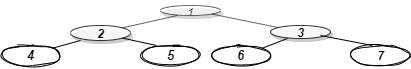``````1 is the root node, so its horizontal distance = 0.
Since 2 lies to the left of 0, its horizontal distance = 0-1= -1
3 lies to the right of 0, its horizontal distance = 0+1 = 1
Similarly, horizontal distance of 4 = Horizontal distance of 2 - 1= -1-1=-2
Horizontal distance of 5 = Horizontal distance of 2 + 1=  -1+1 = 0
Horizontal distance of 6 = 1-1 =0
Horizontal distance of 7 = 1+1 = 2

The bottom-most node at a horizontal distance of -2 is 4.
The bottom-most node at a horizontal distance of -1 is 2.
The bottom-most node at a horizontal distance of 0 is 5 and 6. However, 6 is more towards the right, so 6 is included.
The bottom-most node at a horizontal distance of 1 is 3.
The bottom-most node at a horizontal distance of 2 is 7.

Hence, the bottom view would be 4 2 6 3 7
``````
##### Input format :
``````The first line of input contains an integer ‘T’ denoting the number of test cases.
The next ‘T’ lines represent the ‘T’ test cases.

The first line of input contains the elements of the tree in the level order form separated by a single space.
If any node does not have a left or right child, take -1 in its place. Refer to the example below.
``````

Example :

``````Elements are in the level order form. The input consists of values of nodes separated by a single space in a single line. In case a node is null, we take -1 in its place.

For example, the input for the tree depicted in the below image would be :
```````````` 1
2 3
4 -1 5 6
-1 7 -1 -1 -1 -1
-1 -1

Explanation :
Level 1 :
The root node of the tree is 1

Level 2 :
Left child of 1 = 2
Right child of 1 = 3

Level 3 :
Left child of 2 = 4
Right child of 2 = null (-1)
Left child of 3 = 5
Right child of 3 = 6

Level 4 :
Left child of 4 = null (-1)
Right child of 4 = 7
Left child of 5 = null (-1)
Right child of 5 = null (-1)
Left child of 6 = null (-1)
Right child of 6 = null (-1)

Level 5 :
Left child of 7 = null (-1)
Right child of 7 = null (-1)

The first not-null node (of the previous level) is treated as the parent of the first two nodes of the current level. The second not-null node (of the previous level) is treated as the parent node for the next two nodes of the current level and so on.

The input ends when all nodes at the last level are null (-1).
``````
##### Note :
``````The above format was just to provide clarity on how the input is formed for a given tree.

The sequence will be put together in a single line separated by a single space. Hence, for the above-depicted tree, the input will be given as:

1 2 3 4 -1 5 6 -1 7 -1 -1 -1 -1 -1 -1
``````
##### Output format :
``````For each test case, the bottom view of the binary tree will be printed on a separate line with all the nodes included in the bottom view separated by a single space.
``````
##### Constraints :
``````1 <= T <= 100
1 <= N <= 3000
-10^9 <= DATA <= 10^9

Time Limit: 1 sec
``````
• Q3. DFS Traversal

#### Given an undirected and disconnected graph G(V, E), containing 'V' vertices and 'E' edges, the information about edges is given using 'GRAPH' matrix, where i-th edge is between GRAPH[i] and GRAPH[i]. print its DFS traversal.

``````V is the number of vertices present in graph G and vertices are numbered from 0 to V-1.

E is the number of edges present in graph G.
``````
##### Note :
``````The Graph may not be connected i.e there may exist multiple components in a graph.
``````
##### Input Format :
``````The first line of input will contain two Integers V and E, separated by a single space.

From the second line onwards, the next 'E' lines will denote the undirected edge of the graphs.

Every edge is defined by two single space-separated integers 'a' and 'b', which signifies an edge between the vertices 'a' and 'b'.
``````
##### Output Format :
``````The first line of output will contain the size of the connected components.

For every connected component in the graph, print the vertices of the component in the sorted order of the vertex values separated with a single space.

Print each component in on a different line by making sure that the first vertex of each component is also sorted on the vertex values.

A component having a smaller first vertex in sorted order will come first.
``````
##### Constraints :
``````2 <= V <= 10^3
1 <= E <= (5 * (10^3))

Time Limit: 1sec
``````
• Q4. Explain projects.
• Q5. OOPs

Explain oops concepts, dsa, mongodb

### Interview details

Professional and academic backgroundI completed Computer Science Engineering from Jaypee University Of Engineering And Technology. I applied for the job as Software Analyst in HyderabadProposed: Eligibility criteria6.0 cgpa and no backlogs
Cognizant interview Rounds:Round 1
Round type - Online Coding Interview
Round duration - 120 minutes
Round difficulty - Medium
Round description -

First round is on amcat which checks your basics knowledge of verbal, quantitative aptitude basics of engineering.

Round 2
Round type - Online Coding Interview
Round duration - 60 minutes
Round difficulty - Hard
Round description -

It consist three coding questions based on dsa like tree, graph and vectors maximum connection question i remember of graph.

Round 3
Round type - HR Round
Round duration - 55 minutes
Round difficulty - Medium
Round description -

It was a interview both hr plus technical
Questions were asked on:
State merits demerits of PROJECT?
Can it be scaled?
Normal oops concepts and mongodb.

Cognizant interview preparation:Topics to prepare for the interview - Dsa, django, python, Oops, database, mongodb.Time required to prepare for the interview - 4 monthsInterview preparation tips for other job seekers

Tip 1 : Be confident
Tip 2 : Adhere to what you prepared
Tip 3 : Should know your projects thoroughly.

Application resume tips for other job seekers

Tip 1 : should be easy to understand.
Tip 2 : only facts and no exaggeration in skills.

Final outcome of the interviewSelected
View More### Interview Rounds

Technical

HR

Aptitude Test

Assignment

I applied via Walk-in and was interviewed in Nov, 2020.

### Interview details

Cognizant interview Rounds:
Round 1
Round type - Technical

Round 2
Round type - HR

Round 3
Round type - Aptitude Test

Round 4
Round type - Assignment
Cognizant interview preparation:Interview preparation tips for other job seekers - Re-examine and Apply your Past Experience to the IT Industry.
Get Industry Certifications.
Your Degree in Another Field May Be a Huge Asset.
Be Open to Starting at the Bottom.
Don't Forget the Power of Networking.
Teach Yourself Relevant Tech Skills.
Look for Crossover Positions.
Network with the Right People.
Find the Right Internship.
Create an Effective Resume.
View More1

## Jobs at Cognizant### Interview Rounds

Coding Test

Face to Face

I was interviewed in Dec, 2020.

### Interview Questions

• Q1. Sort Array

#### You are given an array consisting of 'N' positive integers where each integer is either 0 or 1 or 2. Your task is to sort the given array in non-decreasing order.

##### Note :
``````1. The array consists of only 3 distinct integers 0, 1, 2.
2. The array is non-empty.
``````
##### Input Format :
``````The first line of the input contains an integer T denoting the number of test cases.

The first line of each test case contains an integer N, denoting the size of the array.

The second line of each test case contains N space-separated integers, representing the elements of the array.
``````
##### Output Format :
``````The only line of output of each test case consists of N integers, denoting the sorted order of elements in the given array.

Print the output of each test case in a separate line.
``````
##### Note :
``````You do not need to print anything, it has already been taken care of. Just implement the given function.
``````
##### Constraints :
``````1 <= T <= 100
1 <= N <= 10^4
0 <= arr[i] <= 2

where arr[i] is the array element at index 'i'.

Time Limit: 1 sec
``````
• Q2. Minimum steps to reach target by a Knight

#### Your task is to find out the minimum steps a Knight will take to reach the target position.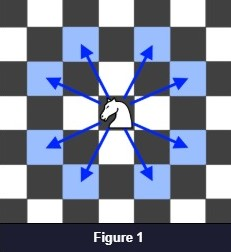##### Example:
``````knightPosition: {3,4}
targetPosition: {2,1}
``````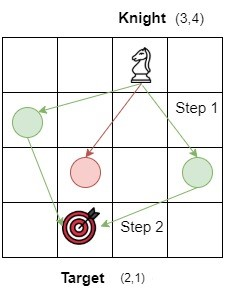``````The knight can move from position (3,4) to positions (1,3), (2,2) and (4,2). Position (4,2) is selected and the ‘stepCount’ becomes 1. From position (4,2), the knight can directly jump to the position (2,1) which is the target point and ‘stepCount’ becomes 2 which is the final answer.
``````

#### Note:

``````1. The coordinates are 1 indexed. So, the bottom left square is (1,1) and the top right square is (N, N).

2. The knight can make 8 possible moves as given in figure 1.

3. A Knight moves 2 squares in one direction and 1 square in the perpendicular direction (or vice-versa).
``````

#### Input format:

``````The first line of input contains an integer ‘T’ denoting the number of test cases.
The next ‘3*T’ lines represent the ‘T’ test cases.

The first line of each test case contains a pair of integers denoting the initial position of the knight.

The second line of each input contains a pair of integers denoting the target position.

The third line of each test case contains an integer ‘N’ denoting the rows/columns of the chessboard.
``````

#### Output format:

``````For each test case, print an integer representing the minimum steps a Knight will take to reach the target position.
``````
##### Note :
``````You do not need to print anything; it has already been taken care of. Just implement the given function.
``````

#### Constraints

``````1 <= T <= 10
1 <= N <= 1000
1 <= KNIGHTPOSITION(X, Y), TARGETPOSITION(X, Y) <= N

Time limit: 1 second
``````
• Q3. Ways To Make Coin Change

#### You are given an infinite supply of coins of each of denominations D = {D0, D1, D2, D3, ...... Dn-1}. You need to figure out the total number of ways W, in which you can make a change for value V using coins of denominations from D. Print 0, if a change isn't possible.

##### Input Format
``````The first line of input contains an integer N, representing the total number of denominations.

The second line of input contains N integers values separated by a single space. Each integer value represents the denomination value.

The third line of input contains the value of V, representing the value for which the change needs to be generated.
``````
##### Output Format:
``````For each test case, print an integer denoting the total number of ways W, in which a change for V is possible.
``````
##### Note:
``````You do not need to print anything, it has already been taken care of. Just implement the given function.
``````

#### Constraints :

``````1 <= N <= 10
1 <= D[i] <=10^5
1 <= V <= 2 * 10^3

Where 'D[i]' represent the value of ith denomination.

Time Limit: 1sec
``````

### Interview details

Professional and academic backgroundI applied for the job as Genc Next in HyderabadProposed: Eligibility criteria6.5 CGPA
Cognizant interview Rounds:Round 1
Round type - Online Coding Interview
Round duration - 120 Minutes
Round difficulty - Medium
Round description -

We have aptitude, reasoning and verbal.

Round 2
Round type - Online Coding Interview
Round duration - 60 minutes
Round difficulty - Easy
Round description -

Round 3
Round type - Face to Face
Round duration - 60 Minutes
Round difficulty - Medium
Round description -

I got the link a day before and my interview was scheduled at 6Pm.

Cognizant interview preparation:Topics to prepare for the interview - C, C++, java, Python, c#.net, sql, some certifications on Machine learningTime required to prepare for the interview - 2 monthsInterview preparation tips for other job seekers

Tip 1 : confidence is most important.
Tip 2 : explaining projects plays a key role.

Application resume tips for other job seekers

Tip 1 : you should be very clear what you have written in your resume.
Tip 2 : projects should be very clear

Final outcome of the interviewSelected
View More1### Interview Rounds

Technical

HR

I applied via Campus Placement and was interviewed in Oct, 2021.

### Interview details

Cognizant interview Rounds:
Round 1
Round type - Technical

Round 2
Round type - HR
Cognizant interview preparation:Interview preparation tips for other job seekers - First of all i want to mention the major things/questions: 1. Introduction 2. Projects 3. Strengths and weaknesses.

Introduction, the way you Introduce yourself is the only main thing, by which you'll be able to route the whole interview in your way.

Project, you need to have full knowledge of all the technologies or domains you've used in your project/projects, and about your role in the project. Because interviewer will going to ask questions in deep.

Strengths and weaknesses, your answer for this question will going to clear so many things for the interviewer, whether he'll hire you or not, as he needs to know that how you'll going to deal with the things while working in the organization, so be smart while answering this question. And take one thing in consideration that, tell your weaknesses which are not relatable to the job role/job profile.

So, the above mentioned questions are important for the interview. And some other things are like, you should've some basic knowledge of programming languages, specially for non CSE/IT students.

At last, all the very best for interviews, hope my experience/ above shared questions will going to help you.

Just be confident.
View MoreContribute to the growing community

Don’t worry. You can choose to be anonymous.